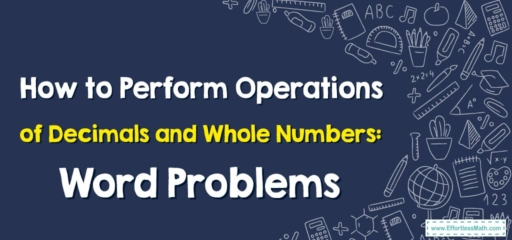# How to Perform Operations of Decimals and Whole Numbers: Word Problems

Hey math learners! Welcome back to another exciting exploration of the world of numbers. Today, we will unravel how to solve word problems involving the operations of decimals and whole numbers. It's important to master these concepts as they're extensively applied in real-world situations. Let's jump right in!## Decimals, Whole Numbers, and Word Problems

A decimal number is a number that contains a decimal point. It represents a whole part and a fraction of a whole. A whole number, on the other hand, is a number without any fractional part, including zero.

Word problems are mathematical problems presented in the form of a story or a real-life scenario. They require you to identify the necessary operations to solve the problem, which could be addition, subtraction, multiplication, or division.

## Step-By-Step Guide to Solving Word Problems Involving Operations of Decimals and Whole Numbers

### Step 1: Understand the Problem

Read the problem carefully and identify what you’re being asked to find. Recognize the key information provided.

### Step 2: Plan the Solution

Decide which mathematical operation(s) you’ll need to use to solve the problem.

### Step 3: Carry Out the Plan

Perform the operation(s). Ensure you place the decimal correctly when dealing with decimal numbers.

### Step 4: Check the Solution

Finally, check your answer. Does it make sense in the context of the problem? If yes, you’ve likely found the correct solution.

### Example:

Let’s try a word problem: Lisa has $$10$$. She bought a pen for $$2.85$$. How much money does Lisa have left?

Step 1: We’re asked to find out how much money Lisa has left after buying a pen.

Step 2: Since she spent money on the pen, we need to subtract the cost of the pen from the amount of money she had.

Step 3: Perform the subtraction: $$10.00\ – 2.85 = 7.15$$. So, Lisa has $$7.15$$ left.

Step 4: Check the answer. Lisa had $$10$$ and spent less than that amount, so it makes sense that she would have over $$7$$ left.

Don’t worry if you don’t get it right away! With practice, solving word problems involving operations of decimals and whole numbers will become second nature. Keep practicing and stay curious!

### What people say about "How to Perform Operations of Decimals and Whole Numbers: Word Problems - Effortless Math: We Help Students Learn to LOVE Mathematics"?

No one replied yet.

X
30% OFF

Limited time only!

Save Over 30%

SAVE $5 It was$16.99 now it is \$11.99